# dr. F.H.C. Bertrand (Fleurianne)

I studied mathematics at the Leibniz University of Hannover (Germany) and obtained my PhD for my work on H(div)-discretizations of two-phase flows in July 2014 under the supervision of Prof. Gerhard Starke.
I then joined the University of Essen for a Post-Doc and started working on solid mechanics, especially as a PI of the projects numerical simulation of thermally induced crack propagationand approximation and reconstruction of the stress tensor in the deformed configuration for hyperelastic materials.
October 2018, I joined the Humboldt-Universität zu Berlin as a W1-professor for Computational Mathematics. There, I broaded my area of expertise in the area of eigenvalues problems.
December 2020, I obtained a tenured position at the Univeristy of Twente as a professor for mathematical theory of the finite element method. In particular, I am the Co-Pi of the research project synthetic data-driven model reduction methods for modal analysis and teaching the scientific computing and the finite element courses.

### Expertise

Engineering & Materials Science
Elasticity
Error Analysis
Mathematics
Eigenvalue Problem
Error Estimator
Finite Element
First-Order System
Least Squares
Linear Elasticity

### Publications

Recent
Bertrand, F., Boffi, D., & Halim, A. (2023). A reduced order model for the finite element approximation of eigenvalue problems. Computer methods in applied mechanics and engineering, 404, Article 115696. https://doi.org/10.1016/j.cma.2022.115696
Bertrand, F., Boffi, D., & Schneider, H. (2023). Discontinuous Petrov-Galerkin Approximation of Eigenvalue Problems. Computational Methods in Applied Mathematics, 23(1), 1-17. Advance online publication. https://doi.org/10.1515/cmam-2022-0069
Alzaben, L. , Bertrand, F., & Boffi, D. (2022). On the Spectrum of an Operator Associated with Least-Squares Finite Elements for Linear Elasticity. Computational Methods in Applied Mathematics, 22(3), 511-528. Advance online publication. https://doi.org/10.1515/cmam-2022-0044
Bertrand, F., & Boffi, D. (2022). First order least-squares formulations for eigenvalue problems. IMA Journal of Numerical Analysis, 42(2), 1339–1363. Advance online publication. https://doi.org/10.1093/imanum/drab005
Bertrand, F., Lambers, L., & Ricken, T. (2021). Least Squares Finite Element Method for Hepatic Sinusoidal Blood Flow. Proceedings in Applied Mathematics and Mechanics, 20(1), Article e202000306. https://doi.org/10.1002/pamm.202000306
Bertrand, F., & Starke, G. (2021). A posteriori error estimates by weakly symmetric stress reconstruction for the Biot problem. Computers & mathematics with applications, 91, 3-16. Advance online publication. https://doi.org/10.1016/j.camwa.2020.10.011
Bertrand, F., Kober, B., Moldenhauer, M., & Starke, G. (2021). Weakly symmetric stress equilibration and a posteriori error estimation for linear elasticity. Numerical Methods for Partial Differential Equations, 37(4), 2783-2802. Advance online publication. https://doi.org/10.1002/num.22741
Bertrand, F., Boffi, D., & Ma, R. (2021). An Adaptive Finite Element Scheme for the Hellinger-Reissner Elasticity Mixed Eigenvalue Problem. Computational Methods in Applied Mathematics, 21(3), 501-512. Advance online publication. https://doi.org/10.1515/cmam-2020-0034
Bertrand, F., & Boffi, D. (2021). Least-squares formulations for eigenvalue problems associated with linear elasticity. Computers & mathematics with applications, 95, 19-27. Advance online publication. https://doi.org/10.1016/j.camwa.2020.12.013
Bertrand, F., Boffi, D., & G. de Diego, G. (2021). Convergence analysis of the scaled boundary finite element method for the Laplace equation. Advances in computational mathematics, 47(3), Article 34. Advance online publication. https://doi.org/10.1007/s10444-021-09852-z
Bertrand, F., Ern, A., & Radu, F. A. (2021). Editorial Robust and reliable finite element methods in poromechanics. Computers & mathematics with applications, 91, 1-2. Advance online publication. https://doi.org/10.1016/j.camwa.2021.04.012
Bertrand, F., Demkowicz, L., & Gopalakrishnan, J. (2021). Recent Advances in Least-Squares and Discontinuous Petrov–Galerkin Finite Element Methods. Computers & mathematics with applications, 95, 1-3. https://doi.org/10.1016/j.camwa.2021.05.029
Alzaben, L. , Bertrand, F., & Boffi, D. (2021). Computation of eigenvalues in linear elasticity with least-squares finite elements: dealing with the mixed system. In F. Chinesta, R. Abgrall, O. Allix, & M. Kaliske (Eds.), 14th World Congress on Computational Mechanics: WCCM-ECCOMAS Congress 2020 (Vol. 700, pp. 1-7). SCIPEDIA. https://doi.org/10.23967/wccm-eccomas.2020.095
Bertrand, F., Boffi, D., Gedicke, J., & Khan, A. (2021). Some remarks on the a posteriori error analysis of the mixed laplace eigenvalue problem. In F. Chinesta, R. Abgrall, O. Allix, & M. Kaliske (Eds.), 14th World Congress on Computational Mechanics: WCCM-ECCOMAS Congress 2020 (Vol. 700, pp. 1-10). SCIPEDIA. https://doi.org/10.23967/wccm-eccomas.2020.314
Bertrand, F., & Schneider, H. (2021). Least-squares methods for linear elasticity: refined error estimates. In F. Chinesta, R. Abgrall, O. Allix, & M. Kaliske (Eds.), 14th World Congress on Computational Mechanics: WCCM-ECCOMAS Congress 2020 (Vol. 800, pp. 1-13). SCIPEDIA. https://doi.org/10.23967/wccm-eccomas.2020.137
Bertrand, F. (2021). A decomposition of the raviart-thomas finite element into a scalar and an orientation-preserving part. In F. Chinesta, R. Abgrall, O. Allix, & M. Kaliske (Eds.), 14th World Congress on Computational Mechanics: WCCM-ECCOMAS Congress 2020 (Vol. 2100). SCIPEDIA. https://doi.org/10.23967/wccm-eccomas.2020.034
Schlottbom, M. , Bertrand, F., & Starke, G. (2021). Towards a metriplectic structure for radiative transfer equations. In Numerical Analysis of Electromagnetic Problems: OWR Workshop Report 2021, 16 https://doi.org/10.14760/OWR-2021-16
Bertrand, F., & Boffi, D. (2020). The Prager–Synge theorem in reconstruction based a posteriori error estimation. In 75 Years of Mathematics of Computation American Mathematical Society. https://doi.org/10.1090/conm/754
Bertrand, F., & Boffi, D. (2020). The Prager-Synge theorem in reconstruction based a posteriori error estimation. In S. C. Brenner, I. Shparlinski, C-W. Shu, & D. B. Szyld (Eds.), 75 Years of Mathematics of Computation: Symposium Celebrating 75 Years of Mathematics of Computation November 1–3, 2018 (Contemporary Mathematics; Vol. 754). American Mathematical Society. https://doi.org/10.1090/conm/754/15151

### Education

CBL award

January 2022: I am very pleased to have been granted within the call for proposals on steps towards challenge-based implementation at the UT, in order to accelerate the adoption of CBL-related principles within the UT.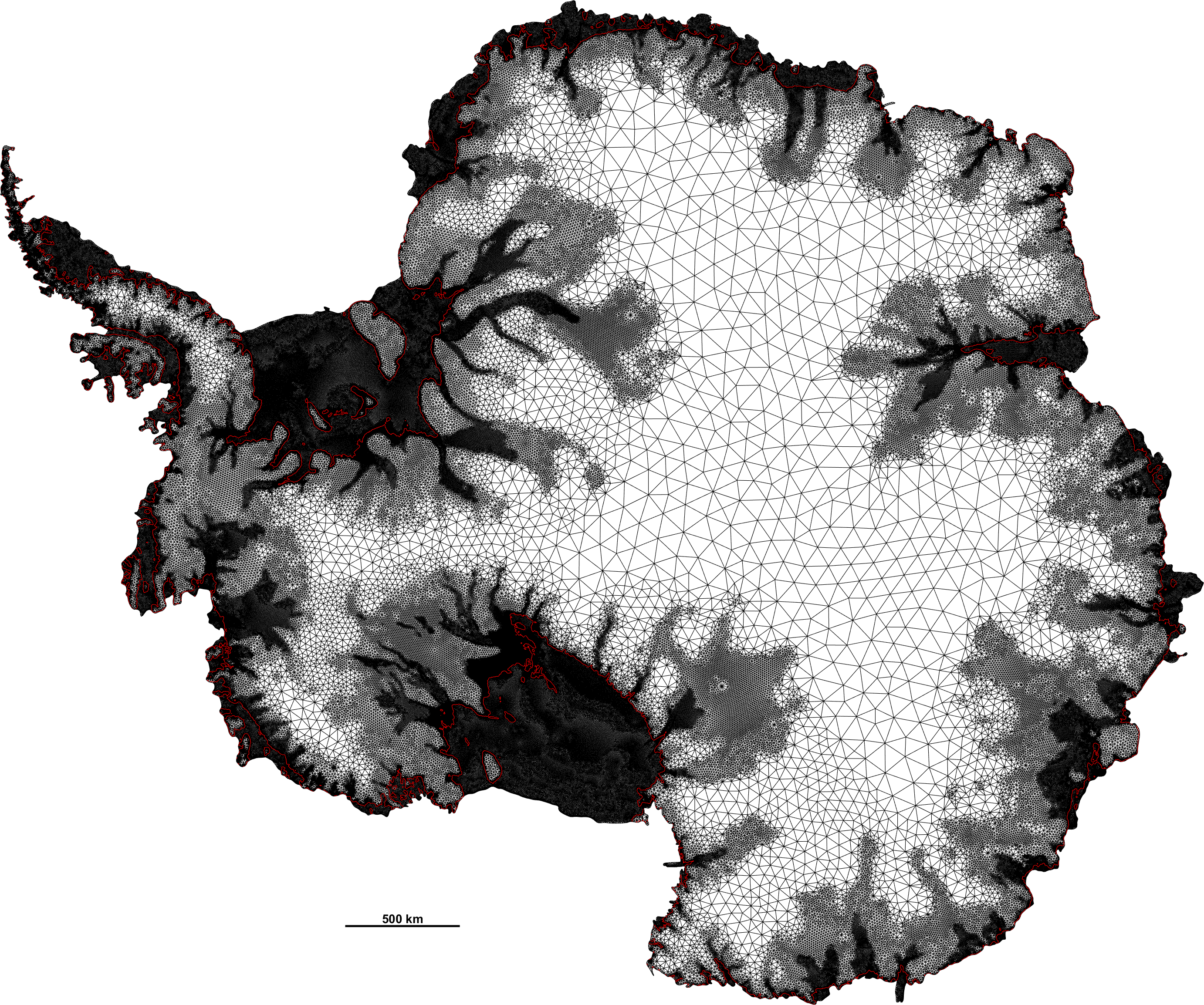Finite element

The finite element method (FEM) is the most widely used method for solving partial differential equations numerically. These equations arise in engineering and mathematical modelling. The range of the applications is immense, although typical applications include solid and fluid mechanics, electromagnetism or biology.
The finite element method is based on a variational equation, for which we will first develop some analytical insight. We will then consider appropriate discrete subspaces (the finite element subspaces) in which we can solve the variational equation. This leads to several algorithms for which the error analysis will be discussed. A further focus will be the design of a corresponding error estimator and its use in an adaptive strategy.

This course has, therefore, two aims:
- Expose the student to the variational framework on which the finite element method is based and enrich the students' knowledge on numerical analysis.
- Practicing programming using Matlab with emphasis on efficiency and correctness.
After this course, the student has the ability to implement the finite element method for a given problem efficiently and reliably.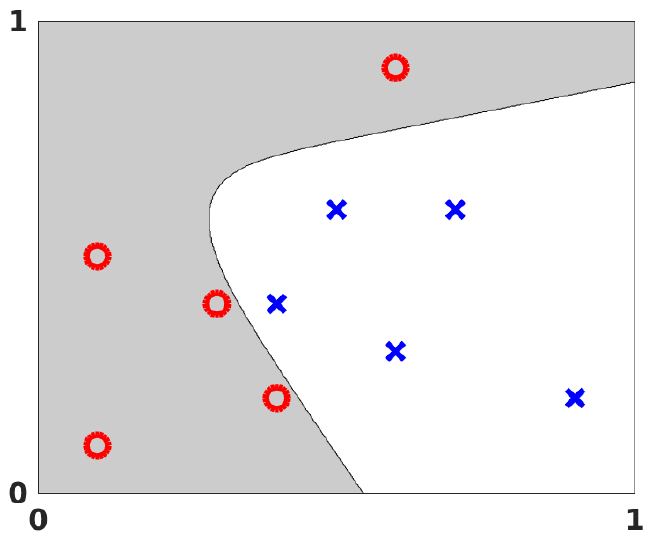Scientific Computing

Thanks to an increase in computing power the opportunities for understanding and solving complex problems in engineering applications, life sciences, environmental issues, urban systems, and finance have rapidly grown in recent decades and thus advanced the corresponding research area Scientific Computing. Typical tasks are modelling the respective problem, developing a numerical method for the approximation, and implementing the respective algorithms on a computer.

In the course Scientific Computing we will look at several (real-world) applications such as determining the eigenfrequencies of a bridge or determining the page rank of a webpage, model them and discuss and implement the respective algorithms to solve the problem in a possibly simplified setting. As solving linear system of equations is a task which appears in many different contexts we start with this topic. As very many phenomena such as the deformation of structures, heat conduction, fluid flow and so on can be modelled well by partial differential equations (PDEs), we discuss PDEs in a simple one-dimensional setting, where we also discuss some modelling aspects and introduce Sobolev spaces. Since the finite element method is by far the most common method to compute an approximation of the solution of an PDE we introduce the Finite Element method still in the simple one-dimensional setting and prove convergence of the approximation towards the solution.
Large-scale eigenvalue problems can be encountered for instance when analyzing social networks or when determining eigenfrequencies of large structures. Therefore, we discuss large scale eigenvalue solvers next. As specific applications we consider PageRank and the Finite Element approximation of the Helmholtz equation in detail. Next, we discuss algorithms from randomized numerical linear algebra which are highly popular in (big-)data analytics and become also more important in numerical simulations. Providing subsequently a brief introduction to deep neural networks with a focus on randomized methods for solving optimization problems gives students basic knowledge about ever more used deep nets that have many different applications. We close the course by considering mixed-integer nonlinear programming problems (MINLP), including linearization-based (outer) approximations and nonlinear branch-and-bound, as well as using solver technology for the practical solution of MINLPs.

### Projects

Third-party projects where I act as PI or Co-Pi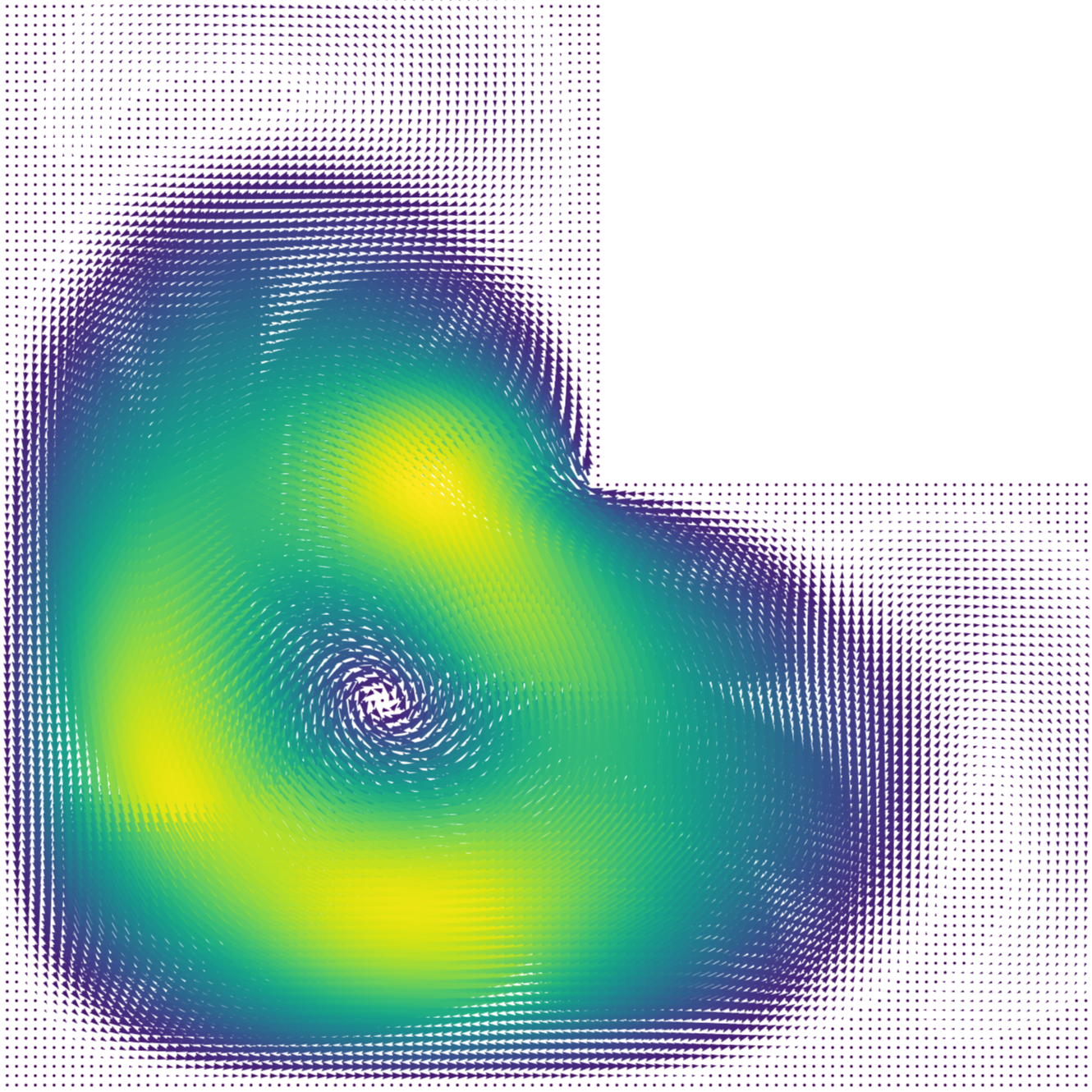Synthetic data-driven model reduction methods for modal analysis

with Prof. Daniele Boffi, founded by KAUST.

We aim at reducing the complexity of the simulations of large, complex systems described in terms of partial differential equations in the spirit of Reduced Order Methods (ROMs).
As a first step, we considered a reduced order method for the approximation of the eigensolutions of the Laplace problem with Dirichlet boundary condition, see our recent preprint here.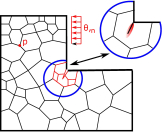Numerical simulation of thermally induced crack propagation

with Prof. Caroline Birk and Prof. Christian Meyer, founded by
Mercator Research Center Ruhr (MERCUR).

Rapid temperature changes may induce thermal stresses, which lead to the initiation and acceleration of damage and fracture processes in structures. The aim of this project is to develop efficient simulation methods for the prediction of thermally-induced crack propagation processes. To this end, a global model based on the scaled boundary finite element method is coupled with a local thermoelastic two-field damage model. Another aim of this project is to develop appropriate error estimators in order to reduce the number of degrees of freedom.
See also our recent publication on the convergence rate of the SBFEM.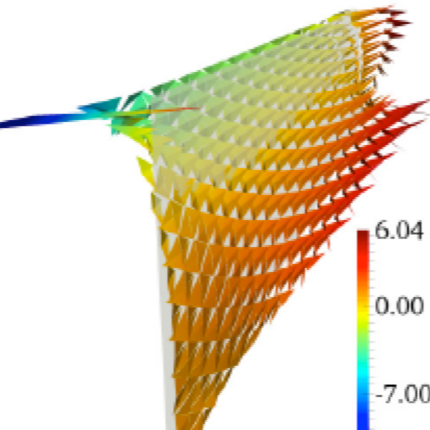Approximation and Reconstruction of the Stresstensor in the deformed configuration for hyperelastic materials

with Prof. Jörg Schröder and Prof. Gerhard Starke, founded in the DFG priority programm SPP 1748.
The main objective of this Priority Programme is the development of modern non-conventional discretisation methods, including the mathematical analysis for geometrically as well as physically non-linear problems in the fields of e.g. incompressibility, anisotropies and discontinuities (cracks, contact).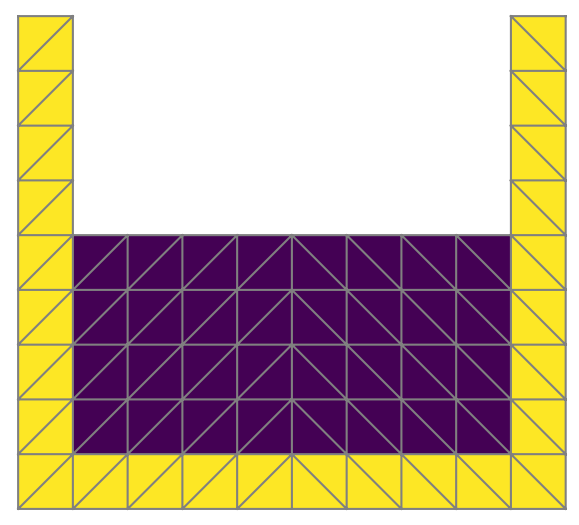Symmetry of Finite Element formulation in solid mechanics

founded by Universität Duisburg-Essen, programm for excellent young researcher.
A common way to determine the displacement of a structure under forces, the so-called primal approach, is based on the minimisation of an energy depending on the displacement variable. However, this so-called primal approach does not lead to uniform accuracy for nearly incompressible materials such as common blood vessel walls or the heart muscle, except if the degree of the polynomial approximation is kept very high, leading to a high computational effort.

In solid mechanics, the quantity expressing the internal forces is the stress. Surely, the stresses can be computed from the primal approach in a post-processing but this approach leads to stresses, which divergence is not squarely integrable. In other words, they are not H(div)-conforming and surface or interface traction forces cannot be evaluated. Moreover, momentum cannot be exactly conserved. As this quantities are driving quantities the standard methods are not accurate enough in many challenging applications and stress-based method are known to be a game changer to obtain guaranteed accuracy. However, in solid mechanics, the symmetric gradient arising in the corresponding partial differential equation is not an easy condition to impose at the discrete level. The conforming discretisation of those stresses, the so-called Arnold-Winter approach, is highly sophisticated and increase the computational cost enormously.
To address the aforementioned issue, my overall aim is to develop numerical methods with very high and guaranteed accuracy. The key objectives are:
• Guaranteed accuracy: given a tolerance, the true error is less and greater than the tolerance, up to multiplicative constants.
• Robustness: the constants in the error bounds don’t depend on model parameter.
• Accessibility : the computational cost is moderate. The constants in the error bounds depend only on local quantities (i.e. mesh-size, shape of the element) and not on global quantities (such that as Poincaré constant of the whole domain)

Further projects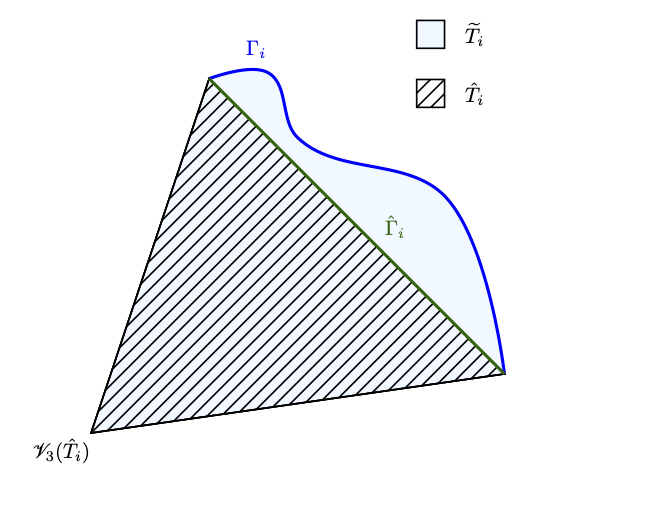Parametric H(div)-conforming elements

Elliptic boundary value problems arising in science and engineering are often posed on domains with curved boundaries. For low-order finite elements it is usually sufficient to use a polygonal approximation of the boundary in order to ensure that the finite element order of convergence is not affected by the inaccurate representation of the boundary. For higher-order finite elements a more accurate representation of the boundary is required which is usually achieved by isoparametric finite elements; i.e., the same finite element space is used once more for the parametrization of a more accurate domain approximation.

Finite element methods which simultaneously approximate scalar (potential) and vector (flux) unknowns are also widely used due to the fact that improved approximation of fluxes is sometimes a desirable goal. Favorable conservation properties or inherent error control are among the advantages of these methods. The treatment of curved boundaries in the context of edge- or face-based finite elements where Neumann boundary conditions are imposed on the normal flux is, however, rather seldom mentioned in the literature. A thorough analysis of the effect of polygonal boundary approximation on the Raviart–Thomas finite element approximations seemed to be missing and was the purpose of this project.

Related publications:

Parametric Raviart-Thomas elements for mixed methods on domains with curved surfaces
Bertrand, F. & Starke, G., 2016, In: SIAM Journal on Numerical Analysis. 54, 6, p. 3648-3667 20

First-order system least squares on curved boundaries: Lowest-order Raviart-Thomas elements
Bertrand, F., Münzenmaier, S. & Starke, G., 2014, In: SIAM Journal on Numerical Analysis. 52, 2, p. 880-894

First-order system least squares on curved boundaries: Higher-order Raviart-Thomas elements
Bertrand, F., Münzenmaier, S. & Starke, G., 2014, In: SIAM Journal on Numerical Analysis. 52, 6, p. 3165-3180 16 p.

First-order system least-squares for interface problems
Bertrand, F., 2018, In: SIAM Journal on Numerical Analysis. 56, 3, p. 1711-1730 20 p.

### Contact Details

University of Twente
Drienerlolaan 5
7522 NB Enschede
The Netherlands

Navigate to location